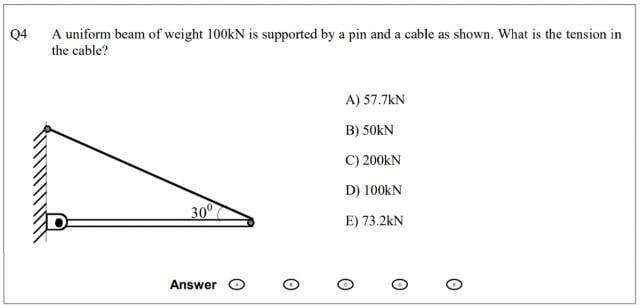# Tension in a Cable

## Homework Statement## Homework Equations

∑MA = 0, where A is the pin.
Tx = Tcos150
Ty = Tsin150

## The Attempt at a Solution

I've drawn in the 100kN force of the beam's weight, and identified that ∑MA = 0. I attempted to find the moment arm, but really wasn't sure how do to it, or if it would even help.

I'm a first year engineering student who's struggling with statics. Any help is appreciated, but please do your best to keep it as easy to understand as possible.

CWatters
Homework Helper
Gold Member

## Homework Statement

View attachment 232980

## Homework Equations

∑MA = 0, where A is the pin.

Tx = Tcos150
Ty = Tsin150

150?

## The Attempt at a Solution

I've drawn in the 100kN force of the beam's weight, and identified that ∑MA = 0. I attempted to find the moment arm, but really wasn't sure how do to it, or if it would even help.

I'm a first year engineering student who's struggling with statics. Any help is appreciated, but please do your best to keep it as easy to understand as possible.

Try summing the torques about A.

The mass of the beam creates a clockwise torque. The wire must create an anticlockwise torque. It's not accelerating so these torques must sum to zero.

Perhaps temporarily replace the diagonal wire with a vertical wire?

150?

Try summing the torques about A.

The mass of the beam creates a clockwise torque. The wire must create an anticlockwise torque. It's not accelerating so these torques must sum to zero.

Perhaps temporarily replace the diagonal wire with a vertical wire?

I replaced the wire with a vertical wire equal to Tsin30, and then wrote that Tsin30-100kN = 0. That means T = 200kN, but I checked the answer to the question, and it's T = 100kN. Have I missed something? I have the moments/torque chapter of my textbook open in front of me, but it's not helping muchgneill
Mentor
Where does the weight of the beam act? If you were to draw its force vector, where would you place it on the diagram?

•CheesyPeeps
Where does the weight of the beam act? If you were to draw its force vector, where would you place it on the diagram?

Thank you so much!! I got it!
I drew the weight's force vector down from the middle of the beam, and when I did that, it finally clicked that the moment arm of the weight would be half the moment arm of Tsin30. I had been stressing over this question since yesterday - I'm so glad I understand it now. Thank you again!

•gneill
CWatters
Yeah, I see that now. I wasn't thinking at all about the moment arm, but once I spotted the fact that Tsin30 and the weight have different moment arms, I got it. Thank you again for your help! Statics has been driving me mad, so I'm really glad I understand this now